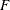# The GLMPOWER Procedure

### Displayed Output

If you use the PLOTONLY option in the PROC GLMPOWER statement, the procedure displays only graphical output. Otherwise, the displayed output of the GLMPOWER procedure includes the following:

• the Fixed Scenario Elements table, which shows all applicable single-valued analysis parameters, in the following order: the dependent variable that represents the cell means scenario (for a univariate model) or the dependent variable transformation (for a multivariate model), the source of the test (that is, the model effect or between-subject contrast), the weight variable, parameters that are input explicitly, parameters that are supplied with defaults, and ancillary results

• an output table that shows the following when applicable (in order): the index of the scenario, the dependent variable that represents the cell means scenario (for a univariate model) or the dependent variable transformation (for a multivariate model), the type of the test, the source of the test (that is, the model effect or between-subject contrast), all multivalued input, ancillary results, the primary computed result, and error descriptions

• plots (if requested)

The exception to these ordering conventions is that the DEPENDENT and EFFECTS= options can be used along with the OUTPUTORDER=SYNTAX or OUTPUTORDER=REVERSE option in the POWER statement to specify the relative location of the output for dependent variable and type and source of test.

Ancillary results include the following:

• Actual Power, the achieved power, if it differs from the input (Nominal) power value

• Actual Alpha, the achieved significance level, if it differs from the input (Nominal) alpha value

• fractional sample size, if the NFRACTIONAL option is used in the POWER statement

• test or numerator degrees of freedom in the test’s critical value

• error or denominator degrees of freedom in the test’s critical value

• Effect, the combination of the within-subject Transformation contrast and between-subject Source test or contrast in a multivariate model

If sample size is the result parameter and the NFRACTIONAL option is used in the POWER statement, then both Fractional and Ceiling sample size results are displayed. Fractional sample sizes correspond to the Nominal values of power. Ceiling sample sizes are simply the fractional sample sizes rounded up to the nearest integer; they correspond to Actual values of power.

The noncentrality parameter is computed and stored in a hidden column called Noncentrality in the Output table. If a univariate test for a multivariate model is specified (that is, one of MTEST=BOX, MTEST=GG, MTEST=HF, or MTEST=UNCORR), then the numerator and denominator degrees of freedom that are used in the noncentralapproximation of the test statistic distribution are computed and stored in hidden columns called NumNCDF and DenNCDF, respectively, in the Output table. These are the only tests for which the degrees of freedom in the noncentralapproximation of the test statistic are different from those in the critical value.